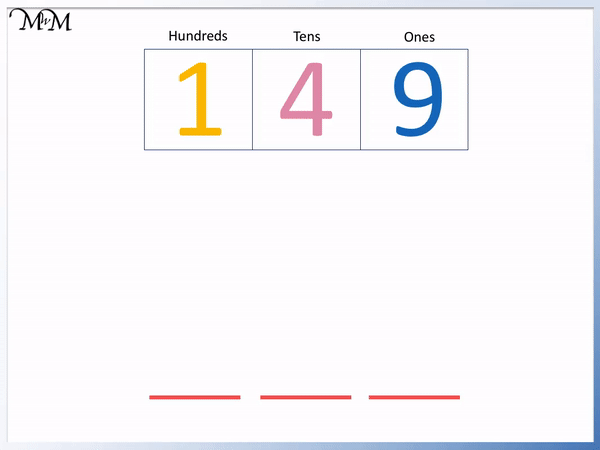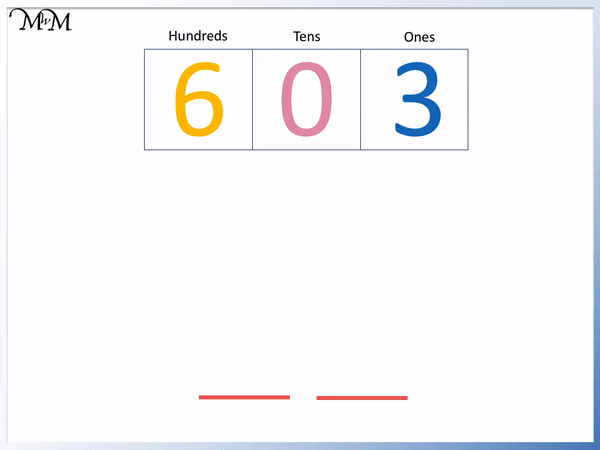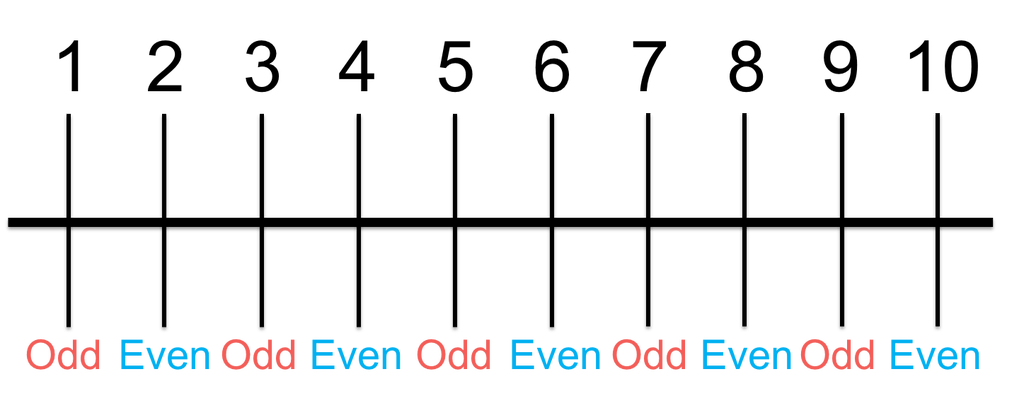# Writing Numbers in Expanded Form

Writing Numbers in Expanded Form• Writing a number in expanded form means to show the value of each digit in the number.
• We start in the ones column.
• 9 is simply 9 ones so we just write 9.
• 4 is in the tens column so is worth 40.
• 1 is in the hundreds column so is worth 100.
• We write 149 in expanded form as 100 + 40 + 9.
• We write the values as a sum.

Writing a number in expanded form means to show the value of each digit in the number.

We write each of these values added together.• To write 79020 in expanded form we show the value of each digit in the number.
• Because the digit of 0 is not worth anything, we do not write it.
• The next smallest digit after zero is 2.
• 2 is in the tens column and is worth 20.
• The next digit in the hundreds column is 0 and so, we do not write it.
• The next digit in the thousands column is 9, which is worth 9000.
• Finally, we have a 7 in the ten-thousands column, which is worth 70000.
• 79020 is written in expanded form as 70000 + 9000 + 20# Writing Numbers in Expanded Form

## What is Expanded Form

Writing a number in expanded form means to write the value of each digit in the number. The number is written as the sum of the separate place values of each digit.

For example 70 is written in expanded form as 70 + 2.72 is made of two digits: 7 and 2.

The 7 is in the tens column and is worth 70. The 2 is in the ones column and is worth 2.

## How to Write a Number in Expanded Form

To write a number in expanded form write the value of each non-zero digit in the number with addition signs between them.

The amount of numbers added together in the expanded form answer will be the same of the amount of non-zero digits in the original number.

Here is an example of writing the number 149 in expanded form.

We have 3 non-zero digits, which are 1, 4 and 9. This means that our expanded form answer will be made up of three numbers added together.The value of each digit is equal to the digit multiplied by the value of the place value column it is in.

The 9 is in the ones column and 9 × 1 = 9. We just write 9.

The 4 is in the tens column and 4 × 10 = 40. The 4 is worth 40.

The 1 is in the hundreds column and 1 × 100 = 100. Therefore this 1 is worth 100.

149 is written in expanded form as 100 + 40 + 9.

The values of the digits are written in the same order as the original number and they all have addition signs between them.

For whole numbers, we can also see that the value of each digit is the same as taking the digit and replacing the digits to the right of it in the number with a zero.

For example, to find the value of the digit 1 in 149, replace the 4 and the 9 with zeros. The digit 1 in 149 is worth 100.

In this next example we will write the number 603 in expanded form.

We don’t include any zeros in our number when writing it in expanded form.

The number 603 is made up of just two non-zero digits: 6 and 3.

This means that when we write 603 expanded form it is made up of two numbers added together.The digit 3 is in the ones column and so is just worth 3.

The 0 is not worth anything so we do not write it.

The 6 is in the hundreds column and so it is worth 6 hundred. We can also see this by replacing the digits to the right of 6 in 603 with zeros. The 6 is worth 600.

We write 603 in expanded form as 600 + 3.

In this next example we will write 3255 in expanded form.

3255 is made up of 4 non-zero digits: 3, 2, 5 and 5.

This means that when we write it in expanded form it will be written as the sum of 4 different numbers.Starting with the digits on the right, the 5 is in the ones column and is worth 5.

The next 5 is in the tens column and is worth 50.

The 2 is in the hundreds column and is worth 200.

Finally, the 3 is in the thousands column and is worth 3000.

3255 is written in expanded form as 3000 + 200 + 50 + 5.

In this next example we will write 24718 in expanded form.

There are 5 non-zero digits so the answer will be made up of 5 different numbers.The 8 is in the ones column and is worth 8.

The 1 is in the tens column and is worth 10.

The 7 is in the hundreds column and is worth 700.

The 4 is in the thousands column and is worth 4000.

The 2 is in the ten-thousands column and is worth 20000.

24718 is written in expanded form as 20000 + 4000 + 700 + 10 + 8.

In this example we write 79020 in expanded form.

Remember that we do not write zeros in expanded form.

We have 3 non-zero digits: 7, 9 and 2.The 2 is in the tens column and is worth 20.

The 9 is in the thousands column and is worth 9000.

The 7 is in the ten-thousands column and is worth 70000.

The number 79020 is written in expanded form as 70000 + 9000 + 20.Now try our lesson on What are Odd ad Even Numbers where we learn about odd and even numbers.error: Content is protected !!